## LetsPlayMaths.Com

WELCOME TO THE WORLD OF MATHEMATICS

# Class-8 Polygon

Introduction to Polygon

Types of Polygons

Concave Polygon

Convex Polygon

Regular Polygon

Irregular Polygon

Sum of Angles of a Polygon

Sum of Exterior Angles of a Polygon

Number of Diagonals of a Polygon

Polygon MCQ

Polygon Worksheets

## Introduction to Polygon

A simple closed curve made up of only line segments is known as a polygon. The line segments are called sides of a polygon. The line segments forming a polygon intersect only at end points and each end point is shared by only two-line segments. Figure below shows various types of polygons.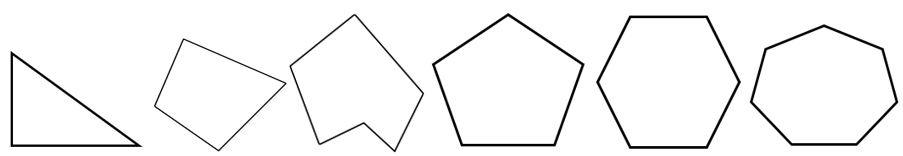Triangle, quadrilateral, pentagon, hexagon, heptagon, octagon, nonagon and decagon can be called as polygon.

## Types of Polygons

There are two types of polygons.

1. Concave Polygon
2. Convex Polygon

## Concave Polygon

A polygon in which at least one angle is greater than 180° is known as a concave polygon. Below given figure is an example of concave polygon, here ∠ABC is more than 180°.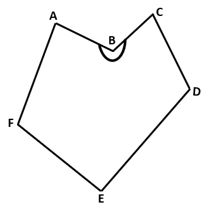## Convex Polygon

A polygon in which each angle is less than 180° is known as a convex polygon. In the below figure ABCD is a convex polygon.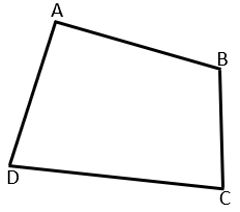## Regular Polygon

A polygon having all sides equal and all angles equal is known as a regular polygon. Equilateral triangle and square are examples of regular polygons.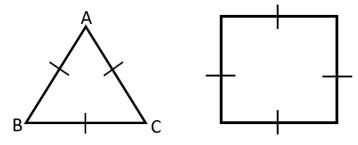## Irregular Polygon

Polygons which do not follow regular polygons properties are known as irregular polygon. Rectangle and rhombus are examples of irregular polygons.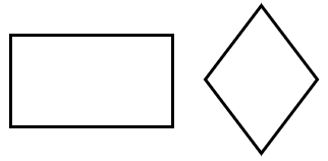## Sum of Angles of a Polygon

If we draw all possible diagonals through a single vertex of a polygon to form as many triangles as possible, then the number of triangles can be formed is two less than the number of sides in the polygon.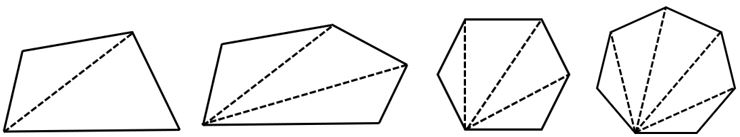So, if a polygon has 'n' sides, then the number of triangle formed will be n − 2.

As we know, the sum of angles of a triangle = 180°

Sum of angles of a polygon having 'n' sides = (n − 2) × 180°

Each interior angle = {(n − 2) × 180°}n

Example 1. Find the measure of each exterior angle of a regular polygon having 9 sides.

Solution. Each exterior angle of a regular polygon having 9 sides = 360°9 = 40°

Example 2. Find the measure of each interior angle of a regular hexagon.
Solution. Each exterior angle of a regular hexagon = 360°6 = 60°

Each interior angles of the hexagon = 180° − 60° = 120°

Example 3. Is it possible to have a regular polygon having each exterior angle 35o.

Solution. Let's assume the regular polygon is having 'n' sides.

Each exterior angle = 360°n

=> 350 = 360°n

=> n = 360°35°

=> n = 1027

Example 4. If each interior angle of a regular ploygon is 156°, then find the number of sides in it.

Solution. Let's assume the number of sides of the regular ploygon is 'n'.

Interior angle = {(n − 2) × 180°}n

=> 156° = (180n − 360)n

=> 156n = 180n − 360

=> 24n = 360

=> n = 36024

=> n = 15

Hence, the regular polygon has 15 sides.

Example 5. An exterior angle and interior angle of a regular polygon are in the ratio 5 : 4. Find the number of sides in the polygon.

Solution. Exterior angle : Interior angle = 5 : 4

In other words, we can say exterior angle is 5x and interior angle is 4x.

As we know, exterior angle + interior angle = 180°

5x + 4x = 180

=> 9x = 180

=> x = 20

Exterior angle = 5x = 100°

Interior angle = 4x = 80°

## Sum of Exterior Angles of a Polygon

If the sides of a polygon are extended in order, the sum of exterior angles formed is always equal to 360°.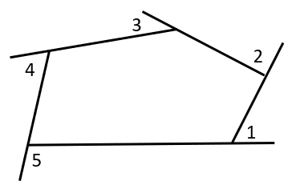∠1 + ∠2 + ∠3 + ∠4 + ∠5 = 360°

## Number of Diagonals of a Polygon

Number of diagonals in a polygon having 'n' sides = n(n − 3)2

Polygon Test - 1

Polygon Test - 2

## Class-8 Polygon Worksheets

Polygon Worksheet - 1

Polygon Worksheet - 2

Polygon Worksheet - 3